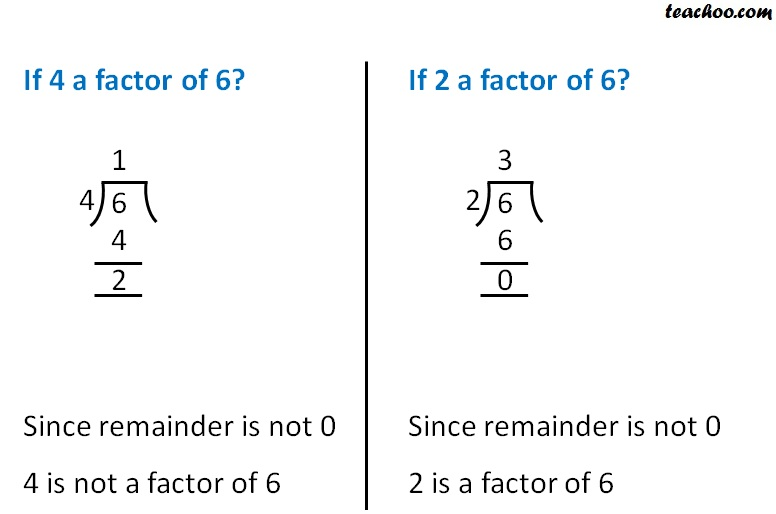If on dividing, remainder is 0

It is factor

Let’s check1. Chapter 3 Class 6 Playing with Numbers
2. Concept wise
3. Factors and Multiples

Transcript

If 4 a factor of 6? Since remainder is not 0 4 is not a factor of 6 If 2 a factor of 6? Since remainder is not 0 2 is a factor of 6

Factors and Multiples